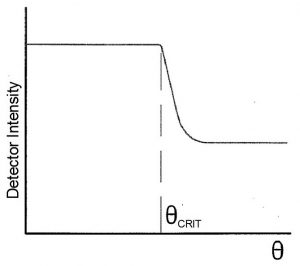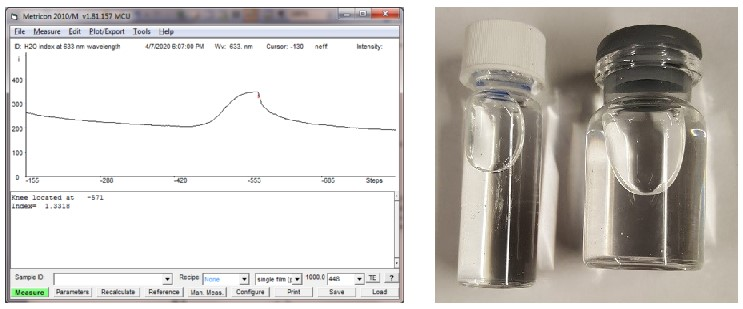Metricon’s Model 2010/M accurately and rapidly measures refractive index of liquids inside sealed containers such as glass vials/bottles, allowing rapid and non-destructive measurement of solution concentration for quality control purposes. Matching liquids are not required between the measuring prism and the wall of the container. If a label is present, the only requirement is that there be a gap of 2-3 mm between the label ends (i.e. the label cannot extend completely around the circumference of the bottle). Excellent results have also been obtained using amber containers and measurements of liquids inside containers of other colours should be possible by appropriate choice of the measuring wavelength.
The principle of measurement is shown below. A container with the liquid to be measured is brought into intimate contact with a measuring prism of index np using a pneumatically-operated coupling head. Collimated laser light is directed onto the base of the prism and the light also refracts into the wall of the container. The prism and container are mounted on a rotary table which can be turned under computer control to change the external angle of incidence θ on the prism so that the laser beam scans through the critical angle for the container-liquid interface:When the beam strikes the container-liquid interface above the critical angle, the light reaching the interface is totally reflected to the photodetector mounted on the opposite side of the prism. However, as the external angle θ changes, θc, the angle in the container wall, drops below the critical angle for the container-liquid interface and some of the light refracts into the liquid causing a sharp drop in the intensity reaching the photodetector. The angular location of this sharp drop (θCRIT ) is easily found by the Model 2010/M’s pattern recognition software:As the light refracts from the prism into the container wall and then into the liquid, from the law of refraction (Snell’s Law) the following equations apply:

nP*sin(θP) = nC*sin(θC) = nL*sin(θL)

By eliminating the middle term, this equation is easily simplified to
nP*sin(θP) = nL*sin(θL)

At the critical angle sin(θL) = 1 and the index of the liquid is
nL = nP*sin(θPCRIT) (1)

Where θPCRIT, (the angle of incidence on the base of the prism at the critical angle for the container-liquid interface), is easily calculated from θCRIT (the external angle on the prism at the critical angle), nP (the prism index), and α (the prism angle).

Equation 1 above shows that the measured index for the liquid is independent of the index of the container wall and depends only on the prism index (which is known to high accuracy).

SPECIFICATIONS
Accuracy, precision, and repeatability:
Sample measurements at a single point on the exterior of a bottle/container are highly repeatable – typically ±.0.0001. However, usable accuracy and precision are both worse (typically ±.0.0005) because of non-parallelism of the container walls which can cause a slight shift in the location of the observed critical angle. The expected accuracy/precision for applications using a specific container type is easily determined by measuring the index variation of a known standard (such as distilled water) for several individual containers at different axial and circumferential locations on the container exterior.

Index range of liquids measurable:
Index of the liquid to be measured must be ~.05 less than the index of the container. Typical index of glass used in bottles/vials is ~1.51 so this spec is easily met by most liquids/solutions.

Measurement wavelength:
The standard wavelength provided with the
Metricon 2010/M system is 637 nm but a different wavelength can be substituted if desired by the user. For most monitoring/quality control applications a single wavelength should be sufficient, but 2010/M systems can also be configured with up to six wavelengths if it is desired to measure at more than
a single wavelength.

Measurement time:
~20 seconds.

[caption id="attachment_6134" align="aligncenter" width="743"]Left: Measurement of DI water index at 633 nm wavelength = 1.3318 (expected value 1.3315) Right: Typical bottles/vials measurable[/caption]

Please contact Lambda Photometrics/Metricon with approximate thickness of silicon layers to be measured to determine which of our four silicon prism types (covering different effective index ranges) are best for your needs. For other very high index (>3.3 ) films, please provide approximate index and thickness and we will advise about measurability.